forsyde-atom-0.3.0.0: Shallow-embedded DSL for modeling cyber-physical systems

ForSyDe.Atom.MoC.SY

Description

The SY library implements the atoms that operate according to the synchronous reactive model of computation. This module also provides a set of helpers for instantiating the MoC layer patterns described in the ForSyDe.Atom.MoC module as meaningful synchronous process constructors.

This module exports a reduced SY language, where all processes are assumed to operate on the same clock rate. For an extended SY language of implicit multi-clock rate systems, check ForSyDe.Atom.MoC.SY.Clocked. Alternatively, this layer can be extended by explicitly wrapping a ForSyDe.Atom.ExB-like layer below it.

Useful pointers:

• ForSyDe.Atom contains general guidelines for using the API
• ForSyDe.Atom.MoC documents details about the internals of the MoC layer, the atoms and the basic structure of all process constructors as MoC patterns.
• ForSyDe.Atom.MoC.SY.Clocked contains an extended SY language of multi-rate clocked systems.
• the naming convention rules on how to interpret the function names based on their number of inputs and outputs.
Synopsis

# Synchronous (SY) event

Acording to the tagged signal model [Lee98] "two events are synchronous if they have the same tag, and two signals are synchronous if all events in one signal are synchronous to an event in the second signal and vice-versa. A system is synchronous if every signals in the system is synchronous to all the other signals in the system." The synchronous (SY) MoC defines no notion of physical time, its tag system suggesting in fact the precedence among events. In simpler words:

The SY MoC
is abstracting the execution semantics of a system where computation is assumed to perform instantaneously (with zero delay), at certain synchronization instants, when data is assumed to be available.

Below is a possible behavior in time of the input and the output signals of a SY process, to illustrate these semantics: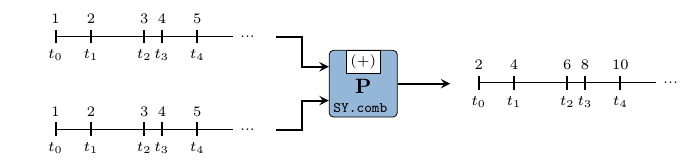The SY tag system is derived directly from the Stream host. Hence a SY Signal is isomorphic to an infinite list, where tags are implicitly defined by the position of events in a signal: $$t_0$$ corresponds with the event at the head of a signal, $$t_1$$ with the following event, etc... The only explicit parameter passed to a SY event constructor is the value it carries (in V). As such, we can state the following particularities:

1. tags are implicit from the position in the Stream, thus they are ignored in the type constructor.
2. the type constructor wraps only a value
3. being a timed MoC, the order between events is interpreted as total.
4. there is no need for an execution context and we can ignore the formatting of functions in ForSyDe.Atom.MoC, thus we can safely assume that $\Gamma\vdash\alpha\rightarrow\beta = \alpha\rightarrow\beta$

newtype SY a Source #

The SY event. It defines a synchronous signal.

Constructors

 SY Fieldsval :: avalue wrapped by the SY event constructor.

#### Instances

Instances details
 Source # Allows for mapping of functions on a SY event. Instance detailsDefined in ForSyDe.Atom.MoC.SY.Core Methodsfmap :: (a -> b) -> SY a -> SY b #(<\$) :: a -> SY b -> SY a # Source # Allows for lifting functions on a pair of SY events. Instance detailsDefined in ForSyDe.Atom.MoC.SY.Core Methodspure :: a -> SY a #(<*>) :: SY (a -> b) -> SY a -> SY b #liftA2 :: (a -> b -> c) -> SY a -> SY b -> SY c #(*>) :: SY a -> SY b -> SY b #(<*) :: SY a -> SY b -> SY a # Source # Implenents the execution semantics for the SY MoC atoms. Instance detailsDefined in ForSyDe.Atom.MoC.SY.Core Associated Typestype Fun SY a b Source #type Ret SY b Source # Methods(-.-) :: Fun SY a b -> Stream (SY a) -> Stream (SY b) Source #(-*-) :: Stream (SY (Fun SY a b)) -> Stream (SY a) -> Stream (SY b) Source #(-*) :: Stream (SY (Ret SY b)) -> Stream (SY b) Source #(-<-) :: Stream (SY a) -> Stream (SY a) -> Stream (SY a) Source #(-&-) :: Stream (SY a) -> Stream (SY a) -> Stream (SY a) Source # Read a => Read (SY a) Source # Reads the value wrapped Instance detailsDefined in ForSyDe.Atom.MoC.SY.Core MethodsreadsPrec :: Int -> ReadS (SY a) #readList :: ReadS [SY a] #readPrec :: ReadPrec (SY a) # Show a => Show (SY a) Source # Shows the value wrapped Instance detailsDefined in ForSyDe.Atom.MoC.SY.Core MethodsshowsPrec :: Int -> SY a -> ShowS #show :: SY a -> String #showList :: [SY a] -> ShowS # Plottable a => Plot (Signal a) Source # SY signals. Instance detailsDefined in ForSyDe.Atom.Utility.Plot Methodssample :: Float -> Signal a -> Samples Source #takeUntil :: Float -> Signal a -> Signal a Source # type Ret SY b Source # Instance detailsDefined in ForSyDe.Atom.MoC.SY.Core type Ret SY b = b type Fun SY a b Source # Instance detailsDefined in ForSyDe.Atom.MoC.SY.Core type Fun SY a b = a -> b

# Aliases & utilities

These are type synonyms and utilities provided for user convenience. They mainly concern the construction and usage of signals.

type Signal a = Stream (SY a) Source #

Type synonym for a SY signal, i.e. "an ordered stream of SY events"

unit2 :: (a1, a2) -> (Signal a1, Signal a2) Source #

Wraps a (tuple of) value(s) into the equivalent unit signal(s). A unit signal is a signal with one event, i.e. a singleton.

Provided helpers: unit, unit2, unit3, unit4.

signal :: [a] -> Signal a Source #

Transforms a list of values into a SY signal.

Reads a signal from a string. Like with the read function from Prelude, you must specify the type of the signal.

>>> readSignal "{1,2,3,4,5}" :: Signal Int
{1,2,3,4,5}


# SY process constuctors

These are specific implementations of the atom patterns defined in ForSyDe.Atom.MoC.

## Simple

Arguments

 :: a initial value -> Signal a input signal -> Signal a output signal

The delay process "delays" a signal with one event. Instantiates the delay pattern.

>>> let s = signal [1,2,3,4,5]
>>> delay 0 s
{0,1,2,3,4,5}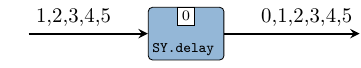Arguments

 :: (a1 -> a2 -> (b1, b2)) function on values -> Signal a1 first input signal -> Signal a2 second input signal -> (Signal b1, Signal b2) two output signals

comb processes map combinatorial functions on signals and take care of synchronization between input signals. It implements the comb pattern (see comb22).

Constructors: comb[1-4][1-4].

>>> let s1 = signal [1..]
>>> let s2 = signal [1,1,1,1,1]
>>> comb11 (+1) s2
{2,2,2,2,2}
>>> comb22 (\a b-> (a+b,a-b)) s1 s2
({2,3,4,5,6},{0,1,2,3,4})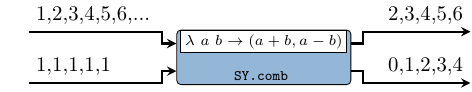Arguments

 :: Signal (a1 -> a2 -> (b1, b2)) signal carrying functions -> Signal a1 first input signal carrying arguments -> Signal a2 second input signal carrying arguments -> (Signal b1, Signal b2) two output signals

reconfig creates an synchronous adaptive process where the first signal carries functions and the other carry the arguments. It imlements the reconfig pattern (see reconfig22).

Constructors: reconfig[1-4][1-4].

>>> let sf = signal [(+1),(*2),(+1),(*2),(+1),(*2),(+1)]
>>> let s1 = signal [1..]
>>> reconfig11 sf s1
{2,4,4,8,6,12,8}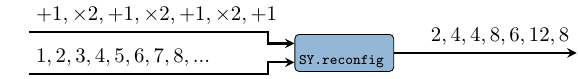Arguments

 :: (b1, b2) values to be repeated -> (Signal b1, Signal b2) generated signals

A signal generator which keeps a value constant. It implements rgw stated0X pattern (see stated22).

Constructors: constant[1-4].

>>> let (s1, s2) = constant2 (1,2)
>>> takeS 3 s1
{1,1,1}
>>> takeS 5 s2
{2,2,2,2,2}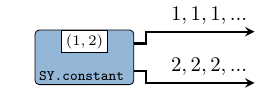Arguments

 :: (b1 -> b2 -> (b1, b2)) function to generate next value -> (b1, b2) kernel values -> (Signal b1, Signal b2) generated signals

A signal generator based on a function and a kernel value. It implements the stated0X pattern (check stated22).

Constructors: generate[1-4].

>>> let (s1,s2) = generate2 (\a b -> (a+1,b+2)) (1,2)
>>> takeS 5 s1
{1,2,3,4,5}
>>> takeS 7 s2
{2,4,6,8,10,12,14}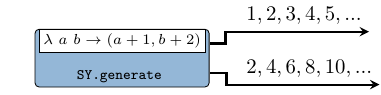Arguments

 :: (b1 -> b2 -> a1 -> a2 -> (b1, b2)) next state function -> (b1, b2) initial state values -> Signal a1 first input signal -> Signal a2 second input signal -> (Signal b1, Signal b2) output signals

stated is a state machine without an output decoder. It implements the stated pattern (see stated22).

Constructors: stated[1-4][1-4].

>>> let s1 = signal [1,2,3,4,5]
>>> stated11 (+) 1 s1
{1,2,4,7,11,16}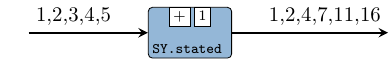Arguments

 :: (b1 -> b2 -> a1 -> a2 -> (b1, b2)) next state function -> (b1, b2) initial state values -> Signal a1 first input signal -> Signal a2 second input signal -> (Signal b1, Signal b2) output signals

state is a state machine without an output decoder. It implements the stated pattern (see state22).

Constructors: state[1-4][1-4].

>>> let s1 = signal [1,2,3,4,5]
>>> state11 (+) 1 s1
{2,4,7,11,16}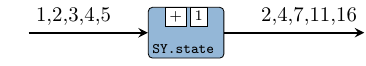Arguments

 :: (st -> a1 -> a2 -> st) next state function -> (st -> (b1, b2)) output decoder -> st initial state -> Signal a1 -> Signal a2 -> (Signal b1, Signal b2)

moore processes model Moore state machines. It implements the moore patterns (see moore22).

Constructors: moore[1-4][1-4].

>>> let s1 = signal [1,2,3,4,5]
>>> moore11 (+) (+1) 1 s1
{2,3,5,8,12,17}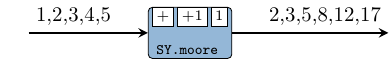Arguments

 :: (st -> a1 -> a2 -> st) next state function -> (st -> a1 -> a2 -> (b1, b2)) outpt decoder -> st initial state -> Signal a1 -> Signal a2 -> (Signal b1, Signal b2)

mealy processes model Mealy state machines. It implements the mealy pattern (see mealy22).

Constructors: mealy[1-4][1-4].

>>> let s1 = signal [1,2,3,4,5]
>>> mealy11 (+) (-) 1 s1
{0,0,1,3,6}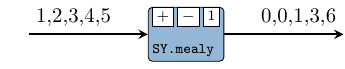# Interfaces

Arguments

 :: (Num t, Ord t, Eq t) => Signal t SY signal carrying DE timestamps -> Signal a first input SY signal -> Signal b second input SY signal -> (SignalBase t a, SignalBase t b) two output DE signals

Wraps explicit timestamps to a (set of) SY signal(s), rendering the equivalent synchronized DE signal(s).

Constructors: toDE, toDE2, toDE3, toDE4.

>>> let s1 = SY.signal [0,3,4,6,9] :: SY.Signal TimeStamp
>>> let s2 = SY.signal [1,2,3,4,5]
>>> toDE s1 s2
{1@0s,2@3s,3@4s,4@6s,5@9s}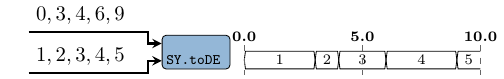Arguments

 :: Signal a SY signal -> Signal b SY signal -> (Signal a, Signal b) SDF signals

Transforms a (set of) SY signal(s) into the equivalent SDF signal(s). The only change is the event consructor, meaning that the order is preserved.

Constructors: toSDF[1-4].

>>> let s = SY.signal [1,2,3,4,5]
>>> toSDF1 s
{1,2,3,4,5}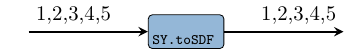Arguments

 :: (Prod, Prod) production rates $$p_1,p_2$$ -> Signal (Vector a) SY signal of vectors of length $$p_1$$ -> Signal (Vector b) SY signal of vectors of length $$p_2$$ -> (Signal a, Signal b) SDF signals where the vectors are serialized.

Transforms a (set of) SY signal(s) of vectors of the same length into equivanlent SDF signal(s) by serializing the vectors according to a production rate. If the production rate and the vector lengths do not match then a runtime error is thrown.

Constructors: toSDF[1-4]'.

>>> let s = read "{<1,2>,<3,4>,<5,6>}" :: SY.Signal (V.Vector Int)
>>> toSDF1' 2 s
{1,2,3,4,5,6}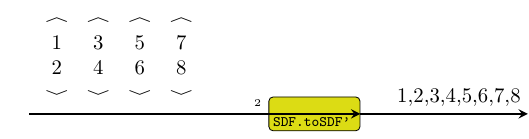zipx :: Vector (Signal a) -> Signal (Vector a) Source #

Synchronizes all the signals contained by a vector and zips them into one signal of vectors. It instantiates the zipx skeleton.

>>> let s1 = SY.signal [1,2,3,4,5]
>>> let s2 = SY.signal [11,12,13,14,15]
>>> let v1 = V.vector [s1,s1,s2,s2]
>>> v1
<{1,2,3,4,5},{1,2,3,4,5},{11,12,13,14,15},{11,12,13,14,15}>
>>> zipx v1
{<1,1,11,11>,<2,2,12,12>,<3,3,13,13>,<4,4,14,14>,<5,5,15,15>}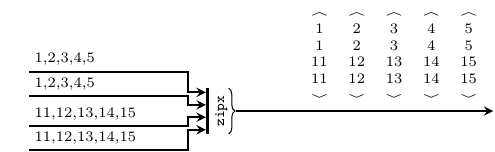unzipx :: Integer -> Signal (Vector a) -> Vector (Signal a) Source #

Unzips the vectors carried by a signal into a vector of signals. It instantiates the unzipx skeleton. To avoid infinite recurrence, the user needs to provide the length of the output vector.

>>> let v1 = V.vector [1,2,3,4]
>>> let s1 = SY.signal [v1,v1,v1,v1,v1]
>>> s1
{<1,2,3,4>,<1,2,3,4>,<1,2,3,4>,<1,2,3,4>,<1,2,3,4>}
>>> unzipx 4 s1
<{1,1,1,1,1},{2,2,2,2,2},{3,3,3,3,3},{4,4,4,4,4}>unzipx' :: Signal (Vector a) -> Vector (Signal a) Source #

Same as unzipx, but "sniffs" the first event to determine the length of the output vector. Has an unsafe behavior!

>>> let v1 = V.vector [1,2,3,4]
>>> let s1 = SY.signal [v1,v1,v1,v1,v1]
>>> s1
{<1,2,3,4>,<1,2,3,4>,<1,2,3,4>,<1,2,3,4>,<1,2,3,4>}
>>> unzipx' s1
<{1,1,1,1,1},{2,2,2,2,2},{3,3,3,3,3},{4,4,4,4,4}>